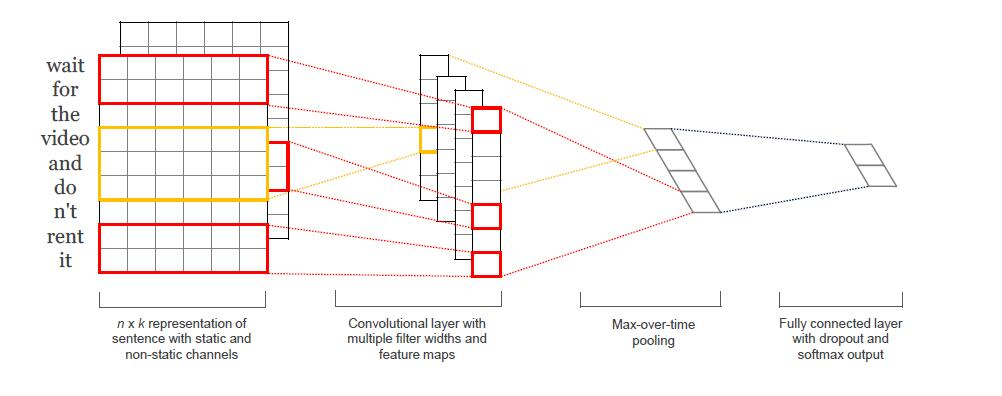# [转]Tensorflow实现的CNN文本分类

## 1. 数据和预处理

1. 从原始数据文件中加载正负向情感的句子。
2. 使用与原始文献相同的代码清理文本数据。
3. 将每个句子加到最大句子长度(59)。我们向所有其他句子添加特殊的操作，使其成为59个字。填充句子相同的长度是有用的，因为这样就允许我们有效地批量我们的数据，因为批处理中的每个示例必须具有相同的长度。
4. 构建词汇索引，并将每个单词映射到0到18,765之间的整数（词库大小）。 每个句子都成为一个整数向量。

## 2. 模型1. 我们不会对我们的词嵌入使用预先训练的word2vec向量。 相反，我们从头开始学习嵌入。
2. 我们不会对权重向量执行L2规范约束。 A Sensitivity Analysis of (and Practitioners’ Guide to) Convolutional Neural Networks for Sentence Classification 发现约束对最终结果几乎没有影响。
3. 原始实验用两个输入数据通道 - 静态和非静态字矢量。 我们只使用一个通道。

## 3. 代码实现

```  ```import tensorflow as tf
import numpy as np

class TextCNN(object):
def __init__(self,sequence_length, num_classes, vocab_size,
embedding_size, filter_sizes, num_filters):
``````

• sequence_length - 句子的长度。注意：我们将所有句子填充到相同的长度（我们的数据集为59）。
• num_classes - 输出层中的类数，在我们的例子中为正（负）。
• vocab_size - 我们的词汇量的大小。 这需要定义我们的嵌入层的大小，它将具有[vocabulary_size，embedding_size]的形状。
• embedding_size - 嵌入的维度。
• filter_sizes - 我们想要卷积过滤器覆盖的字数。 我们将为此处指定的每个大小设置num_filters。 例如，[3，4，5]意味着我们将有一个过滤器，分别滑过3，4和5个字，总共有3 * num_filters过滤器。
• num_filters - 每个过滤器大小的过滤器数量（见上文）。

### 3.1 INPUT PLACEHOLDERS

tf.placeholder创建一个占位符变量，当我们在训练集或测试时间执行它时，我们将其馈送到网络。 第二个参数是输入张量的形状：None意味着该维度的长度可以是任何东西。 在我们的情况下，第一个维度是批量大小，并且使用“None”允许网络处理任意大小的批次。

### 3.2 EMBEDDING LAYER

```  ```with tf.device('/cpu:0'), tf.name_scope("embedding"):
W = tf.Variable(tf.random_uniform([vocab_size,embedding_size],-1.0,1.0),name="W")
self.embedded_chars = tf.nn.embedding_lookup(W,self.input_x)
self.embedded_chars_expanded = tf.expand_dims(self.embedded_chars,-1)
``````

• tf.device（“/ cpu：0”）强制在CPU上执行操作。 默认情况下，TensorFlow将尝试将操作放在GPU上（如果有的话）可用，但是嵌入式实现当前没有GPU支持，并且如果放置在GPU上会引发错误。
• tf.name_scope创建一个名称范围，名称为“embedding”。 范围将所有操作添加到名为“嵌入”的顶级节点中，以便在TensorBoard中可视化网络时获得良好的层次结构。

W是我们在训练中学习的嵌入矩阵。 我们使用随机均匀分布来初始化它。 tf.nn.embedding_lookup创建实际的嵌入操作。 嵌入操作的结果是形状为[None，sequence_length，embedding_size]的三维张量。

TensorFlow的卷积转换操作具有对应于批次，宽度，高度和通道的尺寸的4维张量。 我们嵌入的结果不包含通道尺寸，所以我们手动添加，留下一层shape为[None，sequence_length，embedding_size，1]。

### 3.3 CONVOLUTION AND MAX-POOLING LAYERS

```  ```pooled_outputs = []
for i,filter_size in enumerate(filter_sizes):
with tf.name_scope("conv-maxpool-%s" %filter_size):
# Convolution Layer
filter_shape = [filter_size,embedding_size,1,num_filters]
W = tf.Variable(tf.truncated_normal(filter_shape,stddev=0.1),name="W")
b = tf.Variable(tf.constant(0.1,shape=[num_filters]),name="b")
conv = tf.nn.conv2d(
name="conv"
)
# Apply nonlinearity
# Max-pooling over the outputs
pooled = tf.nn.max_pool(
h,ksize=[1,sequence_length - filter_size +1,1,1],
)
pooled_outputs.append(pooled)

``````
# Combine all the pooled features num_filters_total = num_filters * len(filter_sizes) self.h_pool = tf.concat(3,pooled_outputs) self.h_pool_flat = tf.reshape(self.h_pool,[-1,num_filters_total])

### 3.5 SCORES AND PREDICTIONS

```  ```with tf.name_scope("output"):
W = tf.Variable(tf.truncated_normal([num_filters_total,num_classes],stddev=0.1),name="W")
b = tf.Variable(tf.constant(0.1,shape=[num_classes]),name="b")
self.scores = tf.nn.xw_plus_b(self.h_drop,W,b,name="scores")
self.predictions = tf.argmax(self.scores,1,name="prediction")
``````

### 3.6 LOSS AND ACCURACY

```  ```# Calculate mean cross-entropy loss
with tf.name_scope("loss"):
losses = tf.nn.softmax_cross_entropy_with_logits(self.scores,self.input_y)
self.loss = tf.reduce_mean(losses)
``````

```  ```# Calculate Accuracy
with tf.name_scope("accuracy"):
correct_predictions = tf.equal(self.predictions,tf.argmax(self.input_y,1))
self.accuracy = tf.reduce_mean(tf.cast(correct_predictions,"float"),name="accuracy")
``````

### 3.7 TRAINING PROCEDURE

Graph包含操作和张量。您可以在程序中使用多个Graph，但大多数程序只需要一个Graph。您可以在多个 Session中使用相同的Graph，但在一个 Session中不能使用多Graph。 TensorFlow始终创建一个默认Graph，但您也可以手动创建一个Graph，并将其设置为新的默认Graph，如下图所示。显式创建 Session和Graph可确保在不再需要资源时正确释放资源。

```  ```FLAGS = tf.flags.FLAGS
with tf.Graph().as_default():
session_conf = tf.ConfigProto(
allow_soft_placement=FLAGS.allow_soft_placement,
log_device_placement=FLAGS.log_device_placement
)
sess = tf.Session(config=session_conf)
with sess.as_default():
``````

### 3.8 INSTANTIATING THE CNN AND MINIMIZING THE LOSS

```  ```cnn = TextCNN(
sequence_length=x_train.shape,
num_classes=y_train.shape,
vocab_size=len(vocab_processor.vocabulary)
embedding_size=FLAGS.num_filters,
filter_sizes = map(int, FLAGS.filter_sizes.split(",")),
num_filters = FLAGS.num_filters)
``````

```  ```# Define Training procedure
global_step = tf.Variable(0,name="global_step",trainable=False)
``````

### 3.9 SUMMARIES

TensorFlow有一个概述（summaries），可以在训练和评估过程中跟踪和查看各种数值。 例如，您可能希望跟踪您的损失和准确性随时间的变化。您还可以跟踪更复杂的数值，例如图层激活的直方图。 summaries是序列化对象，并使用SummaryWriter写入磁盘。

```  ```# Output directory for models and summaries
timestamp = str(int(time.time()))
out_dir = os.path.abspath(os.path.join(os.path.curdir, "runs", timestamp))
print("Writing to {}\n".format(out_dir))

``````
# Summaries for loss and accuracy loss_summary = tf.scalar_summary("loss", cnn.loss) acc_summary = tf.scalar_summary("accuracy", cnn.accuracy) # Train Summaries train_summary_op = tf.merge_summary([loss_summary, acc_summary]) train_summary_dir = os.path.join(out_dir, "summaries", "train") train_summary_writer = tf.train.SummaryWriter(train_summary_dir, sess.graph_def) # Dev summaries dev_summary_op = tf.merge_summary([loss_summary, acc_summary]) dev_summary_dir = os.path.join(out_dir, "summaries", "dev") dev_summary_writer = tf.train.SummaryWriter(dev_summary_dir, sess.graph_def)

### 3.10 CHECKPOINTING

```  ```# Checkpointing
checkpoint_dir = os.path.abspath(os.path.join(out_dir, "checkpoints"))
checkpoint_prefix = os.path.join(checkpoint_dir, "model")

``````
# Tensorflow assumes this directory already exists so we need to create it if not os.path.exists(checkpoint_dir): os.makedirs(checkpoint_dir) saver = tf.train.Saver(tf.all_variables())

### 3.11 INITIALIZING THE VARIABLES

```  ```# Initialize all variables
sess.run(tf.global_variables_initializer())
``````

global_variables_initializer函数是一个方便函数，它运行我们为变量定义的所有初始值。也可以手动调用变量的初始化程序。 如果希望使用预先训练的值初始化嵌入，这很有用。

### 3.12 DEFINING A SINGLE TRAINING STEP

```  ```def train_step(x_batch,y_batch):
"""
A single training step
"""
feed_dict = {
cnn.input_x:x_batch,
cnn.input_y:y_batch,
cnn.dropout_keep_prob:FLAGS.dropout_keep_prob
}
_,step,summaries,loss,accuracy = sess.run(
[train_op,global_step,train_summary_op,cnn.loss,cnn.accuracy],feed_dict
)
time_str = datetime.datetime.now().isoformat()
print("{}:step{},loss{:g},acc{:g}".format(time_str,step,loss,accuracy))
``````

feed_dict包含我们传递到我们网络的占位符节点的数据。您必须为所有占位符节点提供值，否则TensorFlow将抛出错误。使用输入数据的另一种方法是使用队列，但这超出了这篇文章的范围。

```  ```def dev_step(x_batch, y_batch, writer=None):
"""
Evaluates model on a dev set
"""
feed_dict = {
cnn.input_x: x_batch,
cnn.input_y: y_batch,
cnn.dropout_keep_prob: 1.0
}
step, summaries, loss, accuracy = sess.run(
[global_step, dev_summary_op, cnn.loss, cnn.accuracy],
feed_dict)
time_str = datetime.datetime.now().isoformat()
print("{}: step {}, loss {:g}, acc {:g}".format(time_str, step, loss, accuracy))
if writer:
``````

### 3.13 TRAINING LOOP

```  ```# Generate batches
batches = data_helpers.batch_iter(
zip(x_train, y_train), FLAGS.batch_size, FLAGS.num_epochs)

``````
# Training loop. For each batch... for batch in batches: x_batch, y_batch = zip(*batch) train_step(x_batch, y_batch) current_step = tf.train.global_step(sess, global_step) if current_step % FLAGS.evaluate_every == 0: print("\nEvaluation:") dev_step(x_dev, y_dev, writer=dev_summary_writer) print("") if current_step % FLAGS.checkpoint_every == 0: path = saver.save(sess, checkpoint_prefix, global_step=current_step) print("Saved model checkpoint to {}\n".format(path))

### 3.14 VISUALIZING RESULTS IN TENSORBOARD

```  ```tensorboard --logdir `/path/`
``````

• 我们的训练指标并不平滑，因为我们使用小批量。 如果我们使用较大的批次（或在整个训练集上评估），我们会得到一个更平滑的蓝线。
• 因为测试者的准确性显着低于训练准确度，我们的网络在训练数据似乎过拟合了，这表明我们需要更多的数据（MR数据集非常小），更强的正则化或更少的模型参数。 例如，我尝试在最后一层为重量添加额外的L2正则，并且能够将准确度提高到76％，接近于原始文献。
• 因为使用了dropout，训练损失和准确性开始大大低于测试指标。

## 4. EXTENSIONS AND EXERCISES

• 使用预先训练的word2vec向量初始化嵌入。 为了能够起作用，您需要使用300维嵌入，并用预先训练的值初始化它们。
• 限制最后一层权重向量的L2范数，就像原始文献一样。 您可以通过定义一个新的操作，在每次训练步骤之后更新权重值。
• 将L2正规化添加到网络以防止过拟合，同时也提高dropout比率。 （Github上的代码已经包括L2正则化，但默认情况下禁用）
• 添加权重更新和图层操作的直方图summaries，并在TensorBoard中进行可视化。

- - Soul Joy Hub

- - V2EX

- - IT瘾-dev

- - IT瘾-tuicool

## 使用 Tensorflow 构建 CNN 进行情感分析实践 - 腾讯云社区 - 腾讯云

- -
Web挖掘中的情感分析类问题，其实是一个分类问题. 而CNN可以用来处理分类任务，就是在最终的softmax函数计算属于各个类的概率，并归属到概率最大的类. 本次实验参照的是Kim Yoon的论文Convolutional Neural Networks for Sentence Classification.

## Zite 正和 CNN 谈判收购事宜？

- Leo - 爱范儿 · Beats of Bits

- - IT瘾-dev